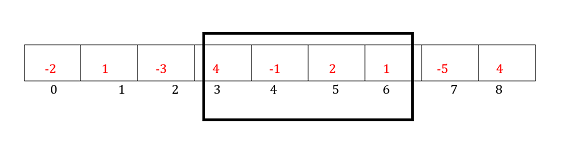# PHP Program for Largest Sum Contiguous Subarray

## What is PHP?

PHP (Hypertext Preprocessor) is a widely used server-side scripting language for web development. It allows developers to embed code within HTML files, enabling the creation of dynamic web pages and interactions with databases. PHP is known for its simplicity, versatility, and extensive integration capabilities with popular databases. It offers a broad range of extensions and has a large community of developers, ensuring ample resources and support.

## PHP Program for Largest Sum Contiguous Subarray4+ (-1) +2 +1 = 6

Maximum contiguous sum is = 6

Kadane's Algorithm is an efficient algorithm used to find the maximum sum of a contiguous subarray within a given array. It was developed by Jay Kadane in 1984.

The algorithm works by iteratively scanning the array and maintaining two variables: max_so_far and max_ending_here. Here's how the algorithm works:

• Initialize max_so_far and max_ending_here variables to the first element of the array or to a minimum value (e.g., PHP_INT_MIN) if the array contains negative numbers.

• Iterate through the array from the second element onwards.

• For each element, update max_ending_here by adding the current element to it.

• If max_ending_here becomes negative, reset it to 0 because including the current element in the subarray will decrease the sum.

• If max_ending_here is greater than max_so_far, update max_so_far with the new maximum sum.

• Repeat steps 3 to 5 for the remaining elements of the array.

• After iterating through the entire array, max_so_far will hold the maximum sum of a contiguous subarray.

• Return max_so_far as the result.

Kadane's Algorithm has a time complexity of O(n), where n is the size of the array, as it only requires a single pass through the array. This makes it an efficient solution for finding the maximum sum contiguous subarray.

### Example

<?php
// PHP program to print largest
// contiguous array sum
function maxSubArraySum($a,$size)
{
$max_so_far = PHP_INT_MIN;$max_ending_here = 0;
for ($i = 0;$i < $size;$i++)
{
$max_ending_here =$max_ending_here + $a[$i];
if ($max_so_far <$max_ending_here)
$max_so_far =$max_ending_here;

if ($max_ending_here < 0)$max_ending_here = 0;
}
return $max_so_far; } // Driver code$a = array(-2, 1, -3, 4, -1, 2, 1, -5, 4);
$n = count($a);
$max_sum = maxSubArraySum($a, $n); echo "Maximum contiguous sum is " ,$max_sum;
?>


### Output

Maximum contiguous sum is 6


### Example

<?php
function maxSubArraySum($a,$size)
{
$max_so_far =$a;
$curr_max =$a;
for ($i = 1;$i < $size;$i++)
{
$curr_max = max($a[$i],$curr_max + $a[$i]);
$max_so_far = max($max_so_far,
$curr_max); } return$max_so_far;
}
// Driver Code
$a = array(-2, 1, -3, 4, -1, 2, 1, -5, 4);$n = sizeof($a);$max_sum = maxSubArraySum($a,$n);
echo "Maximum contiguous sum is " .
$max_sum; ?>  ### Output Maximum contiguous sum is 6  ### Another approach with start and end indexes ### Example <?php // PHP program to print largest // contiguous array sum function maxSubArraySum($a, $size) {$max_so_far = PHP_INT_MIN;
$max_ending_here = 0;$start = 0;
$end = 0;$s = 0;
for ($i = 0;$i < $size;$i++)
{
$max_ending_here +=$a[$i]; if ($max_so_far < $max_ending_here) {$max_so_far = $max_ending_here;$start = $s;$end = $i; } if ($max_ending_here < 0)
{
$max_ending_here = 0;$s = $i + 1; } } echo "Maximum contiguous sum is ".$max_so_far."";
echo "Starting index ". $start . "". "Ending index " .$end . "";
}
// Driver Code
$a = array(-2, 1, -3, 4, -1, 2, 1, -5, 4);$n = sizeof($a);$max_sum = maxSubArraySum($a,$n);
?>


### Output

Maximum contiguous sum is 6
Starting index 3
Ending index 6


## Conclusion

The PHP program for finding the largest sum contiguous subarray utilizes dynamic programming and Kadane's algorithm. The dynamic programming approach is employed to efficiently solve the problem by breaking it down into smaller sub problems and storing the solutions in an array.

Kadane's algorithm is a key component of the program and is responsible for finding the largest sum contiguous subarray. It iterates over the array, continuously updating the current sum by either adding the current element or starting a new subarray. The maximum sum encountered is stored in the \$maxSum variable. The program efficiently handles both positive and negative numbers in the array. It identifies the subarray with the largest sum by keeping track of the start and end indices, allowing for the extraction of the subarray using array_slice.

By utilizing dynamic programming and Kadane's algorithm, the program achieves a time complexity of O(n), where n is the size of the array. This ensures an efficient solution for finding the largest sum contiguous subarray in PHP.

Updated on: 01-Aug-2023

93 Views